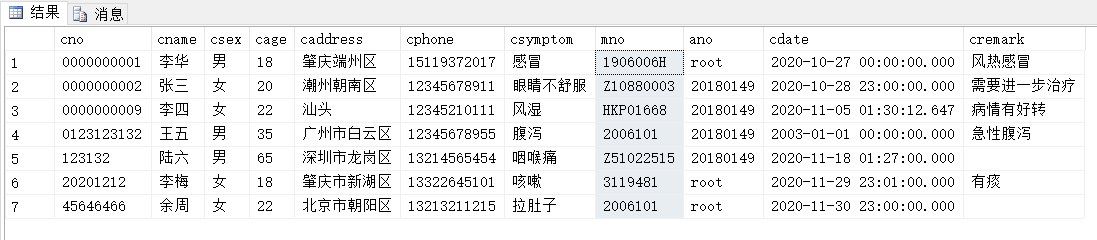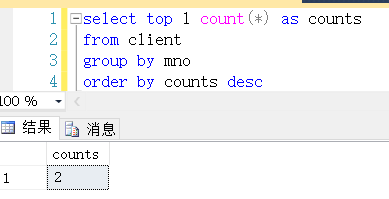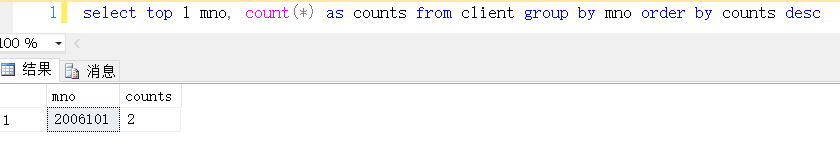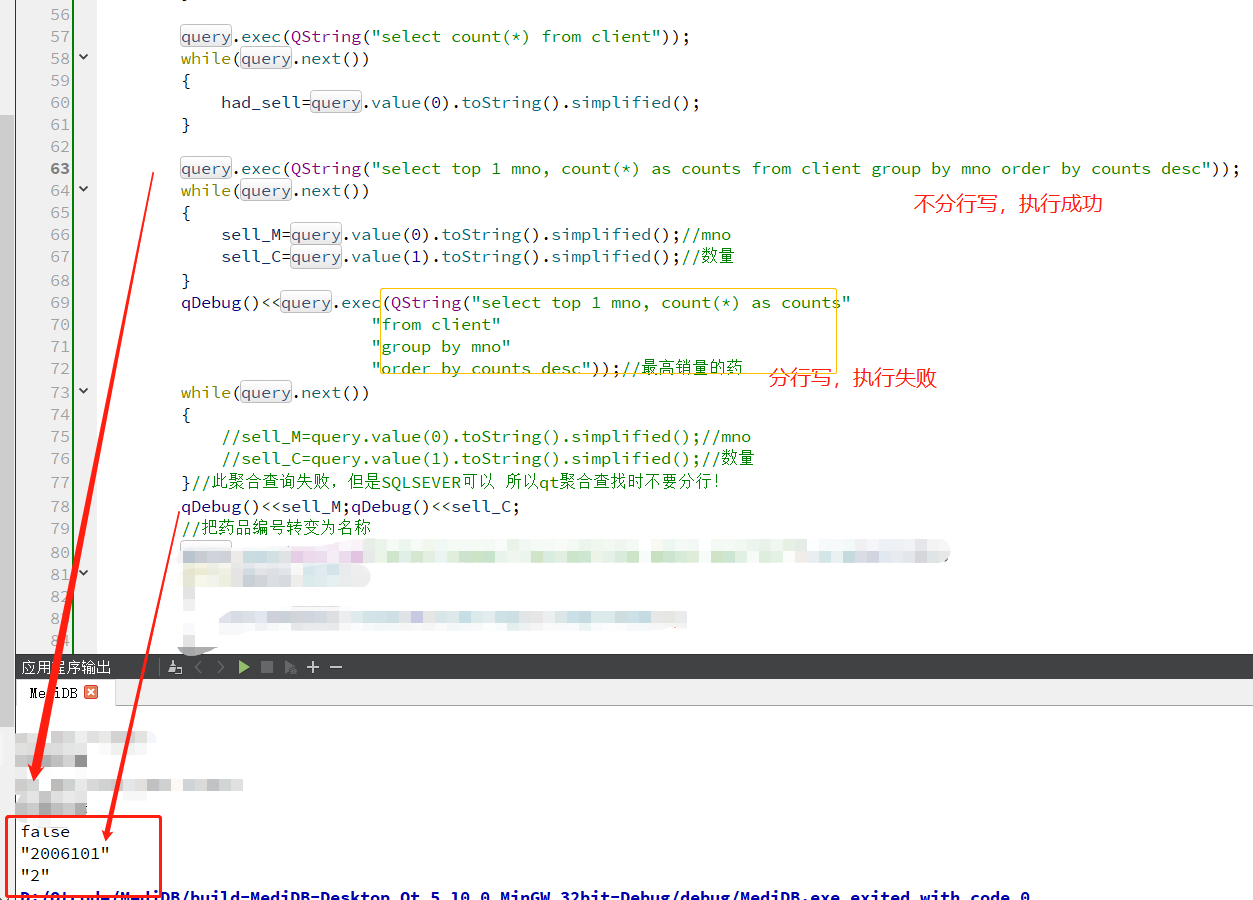• 以下查询语句返回 customer表中 city重复次数（count） 最多的20条记录： SELECT city, count( * ) AS count FROM customer GROUP BY city ORDER BY count DESC LIMIT 20 以下查询语句返回 article_keyword 表中 ...
以下查询语句返回 customer表中 city重复次数（count） 最多的20条记录：
SELECT city, count( * ) AS count
FROM customer
GROUP BY city
ORDER BY count DESC
LIMIT 20
以下查询语句返回 article_keyword 表中 keyword 的重复次数（count） 排名前 6 的数值。通过添加 DISTINCT 返回唯一记录：
SELECT DISTINCT count( * ) AS count
FROM article_keyword
GROUP BY keyword
ORDER BY count DESC
LIMIT 6


展开全文sql
• sql查询出现次数最多的记录的名称和现次数 有一个表：client 需求：查找表中出现次数最多的药品mno名称，和最高销量（出现次数） 1.最高销量： select top 1 count(*) as counts from client group by mno order by...
最近在做一个qt项目，数据库SQL语句这东西，有段时间没用了，然后就会遗忘某些知识。比如聚合查找。
sql查询出现次数最多的记录的名称和现次数
有一个表：client需求：查找表中出现次数最多的药品mno名称，和最高销量（出现次数）
1.最高销量：(排序默认是升序，降序要加DESC)
select top 1 count(*) as counts
from client
group by mno
order by counts desc

查询结果：2.最高销量的药品mno名称
select top 1 mno, count(*) as counts
from client
group by mno
order by counts desc

查询结果：QT聚合查找失败解决
QT中执行区别：我测试后发现后者查不出来，执行语句返回false，前者没有分行的SQL语句就能正确查询出来。
这种莫名错误遇到就记下来了，如果聚合查询失败，但是在SQLSEVER里可以正确执行， 那么在QT里聚合查找时不要分行，否则会执行失败！


展开全文• 自己一直无法解决。想做到查询出，出现最多的中文词汇。这样sql语句应该怎么写group by好像不支持中文吧
• mport java.util.Arrays; import java.util.Collection;...System.out.println(maps.getKey() + "这个字符出现次数最多,共" + obj[obj.length-1] + "个重复"); } } } }
mport java.util.Arrays;
import java.util.Collection;
import java.util.HashMap;
import java.util.Map;
import java.util.Map.Entry;
public class Test {public static void main(String[] args) {String str ="aaaabb555555bccd";HashMap<String, Integer> map = new HashMap<String, Integer>();for(int i=0; i<str.length(); i++){String var = String.valueOf(str.charAt(i));if(!map.containsKey(var)){map.put(var, 1);}else{int aa = map.get(var);map.put(var, aa+1);}}for(Entry<String, Integer> maps : map.entrySet()){System.out.println(maps.getKey()+"的个数:"+maps.getValue());}//排序数组取得最大值Collection<Integer> c = map.values();Object[] obj = c.toArray();Arrays.sort(obj);//map通过value找到keyfor(Entry<String, Integer> maps : map.entrySet()){if(obj[obj.length-1].equals(maps.getValue())){System.out.println(maps.getKey() + "这个字符出现的次数最多,共" + obj[obj.length-1] + "个重复");}}}
}

展开全文• 思路：区间加询问出现次数满足要求的的高度，很明显得主席树的相关操作。有点小模板题的味道。 要找的是满足题目要求高度的最大值，首先看a的范围，先离散化一下，然后对应树存的值就是这个高度出现的次数，只不过这...题意：题目给定一个序列，然后会有m次询问，每次询问让我们找到是否有山峰的高度满足大于(r-l+1)/t，如果有的话输出满足这个条件的山峰最大的高度，否则的话输出-1。
思路：区间加询问出现次数满足要求的的高度，很明显得主席树的相关操作。有点小模板题的味道。
要找的是满足题目要求高度的最大值，首先看a的范围，先离散化一下，然后对应树存的值就是这个高度出现的次数，只不过这是对应离散化数组的，最后还要再转化为原数组的实际高度，wa了好几次。
然后查询函数的时候，因为要找的是最大值，所有我们在递归往下进行的时候先先走右子树，只有右子树不满足条件才会往左走，然后查询函数的初始值是0，如果最后还是0那就说明确实不存在这个点，返回-1即可。
直接上代码了。
#include <bits/stdc++.h>

using namespace std;
typedef long long ll;
const int MAXN = 2e5 + 7;
int n,m,a[MAXN],root[MAXN],size,b[MAXN];

struct node{
int l,r,sum;
}tree[MAXN*60];

void init(){
memset(tree,0,sizeof(tree));
memset(root,0,sizeof(root));
size = 0;
}

void modify(int l,int r,int pre,int &now,int p){
now = ++size;
tree[now] = tree[pre];
tree[now].sum++;
if(l == r) return;
int mid = (l+r)>>1;
if(p <= mid) modify(l,mid,tree[pre].l,tree[now].l,p);
else modify(mid+1,r,tree[pre].r,tree[now].r,p);
}

int query(int now,int pre,int l,int r,int len){
if(l == r){
return l;
}
int mid = (l+r)>>1;
int res = 0,tmp;
if(tree[tree[now].r].sum - tree[tree[pre].r].sum > len){
tmp = query(tree[now].r,tree[pre].r,mid+1,r,len);
if(tmp != -1) res = max(res,tmp);
}
if(tree[tree[now].l].sum - tree[tree[pre].l].sum > len){
tmp = query(tree[now].l,tree[pre].l,l,mid,len);
if(tmp != -1) res = max(res,tmp);
}
if(res == 0) res = -1;
return res;
}

int main()
{
scanf("%d%d",&n,&m);
init();
int cnt = 0;
for(int i = 1;i <= n;i ++){
scanf("%d",&a[i]);
b[++cnt] = a[i];
}
sort(b+1,b+1+n);
int num = unique(b+1,b+1+n)-b-1;
for(int i = 1;i <= n;i ++){
a[i] = lower_bound(b+1,b+1+num,a[i])-b;
}
for(int i = 1;i <= n;i ++){
//scanf("%d",&a[i]);
modify(1,num,root[i-1],root[i],a[i]);
}
int x,y,t;
while(m--){
scanf("%d%d%d",&x,&y,&t);
// if(n == 1){
//       	if(x == 1 && y == 1 && t == 1){
//       		printf("-1\n");
//       		continue;
//       	}
//       }
//printf("%d\n",query(root[y],root[x-1],1,num,(y-x+1)/t));
int id = query(root[y],root[x-1],1,num,(y-x+1)/t);
if(id != -1) printf("%d\n",b[id]);
else printf("-1\n");
}
return 0;
}



展开全文• import java.util.*;... 出现次数 */ public static void main(String[] args){ int charabc=0; int number=0; int space=0; int othen=0; int countLettet=1; HashMap<Character,Integer>.
• （我的程序没有确定N的值，而是将所有单词按出现次数由多到少排序输出到文件中） 二、TXT文件（摘自https://www.lz13.cn/lizhiyingyu/6970.html） You Have Only One Life.txt: 　There are moments ...
• 查询字符串中出现次数最多的字符以及出现次数 最优解： // 查询字符串出现最多的次数 var randomStr = "sssfgtdaddddddddadadadadadddddfssddfsssfss"; function getMax(str) { var newStr = str.split('').sort...
• 主要介绍了通过LinQ查询字符出现次数的实例方法，大家参考使用吧
• 查询某个字段出现的次数：select A,count(*) from tableA group by A; 查询出现次数大于1的：select A,count(*) from tableA group by A having count(*) &gt; 1;
• MySQL查询逗号出现次数： 其中drafts表中有userId，userId以“1,2,3”格式存储。 select (length(userId) - length(replace(userId,',',''))) nums from drafts WHERE userId is not null;mysql
• MySQL查询的方法很多，下面为您介绍的MySQL查询语句用于实现查询重复出现次数最多的记录，对于学习MySQL查询有很好的帮助作用。 在有些应用里面，我们需要查询重复次数最多的一些记录，虽然这是一个很简单的查询语句...
• public void validatePsqlReelDataQuantity() throws SQLException, InstantiationException, IllegalAccessException, ClassNotFoundException { String text = data.get(dataKey); System.out.println("input...
• 其中在实验室测试的时候问题很少，但是在现场Bug频发，在找Bug看日志文件的时候，一些内容出现了好多次，我想统计一下这个情况出现了多少次，于是乎产生了编写一个软件，能够查询文件中的要查询内容出现次数，甚至...Qt C++
• MySQL查询的方法很多，下面为您介绍的MySQL查询语句用于实现查询重复出现次数最多的记录，对于学习MySQL查询有很好的帮助作用。 在有些应用里面，我们需要查询重复次数最多的一些记录，虽然这是一个很简单的查询...oracle mysql 使用频率 count group
• 出现重复的列 其他列 A XXXXXX A XXXXXX B XXXXXX B XXXXXX C XXXXXX D XXXXXX C XXXXXX A XXXXXX 项得到的结果 出现重复的列 重复第N次出现 A 0 A 1 B 0 B 1 C 0 D 0 C 1 A 2 求大神帮忙给个sqloracle sql 数据
• 字段里面包含了不定数量重复的字符串，想查询包含N个字符的记录 select id,name from table where ((length(name )-length(replace(name ,‘abc8’,’’)))/4)>5 上面的4就是字符串的长度，5就是包含字符串 ......